Gravimotion Interpretation of Reality

Newton's inertia laws reveal how mass and kinetic energy that is motion energy make one. The flow of light, which is massless, is also energy on the move given here the name motion energy.
Motion energy does not require mass nor its inertia to be on the move.
In this interpretation, motion is considered to be the fabric of energy; the word motion makes one of the words "energy on the move".
Both physics and gravimotion interpretation of Nature fight their ways toward, among other, a common goal that is unification but travel different paths; in gravimotion a particle matter is not made of mass it is occurring at the image of gravity that is stalled motion energy.
Besides should you decide to read about gravimotion, this Frenchman's english writing is not the best.

Gravimotion's motion-volume. Physics teaches that light rays are occurring as massless particle photons; yet because observed and measured a photon occupies a volume of spacetime, given here the name motion-volume.
A motion-volume is considered to be the implementation of our two mental concepts that are motion and energy.
Unlike an object's volume, which does not involve time, a motion-volume's three dimensions are occurring as a combination of space and time.
And unlike an object's movement, a motion-volume is involving no mass, at the image of light rays or photons on which it is conceived.
The motion-volume's time duration is finite, it is lasting a time duration not exceeding the present, as nothing exists in the past and nothing exists in the future; yet a motion-volume renews itself over and over just as the present does; a motion-volume is at same time finite and infinite, and it obeys Newton's first law: a uniform motion-volume continues unaffected unless modified by collision or overlap by another motion-volume.

The motion-volume mathematical expression.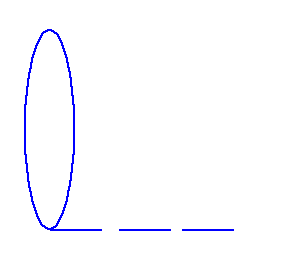In this animation, successive motion-volumes are following each other; each one is a dynamic cylinder, whose volume is the product of the moving cross section by the time it takes to move left to right, a duration represented by the moving horizontal hyphenated line length.
Calling r the radius of the circle, its surface is a distance d squared d2 = r2; the motion-volume is defined as the product d2 . 1 / t2 a squared distance multiplied by the inverse of a squared time, which is a speed d / t squared.
The horizontal line that is defining such volume is the inverse of a squared time duration 1 / t2, which in the following is denoted f2 as f happens to be the letter t flipped upside down or the inverse of it; the ratio d2 / t2 becoming a product d2 . f2 now fitting a 3 dimensional volume unlike a speed odd dimensions.
Finally, the quantity f2 is placed first as it is chosen to be the variable, while d2 the cross section is used as reference, which is the opposite of a speed d / t in which the distance d in meters instead is the variable providing the distance travelled during a reference chosen as 1 second in the SI system. The motion-volume becomes f2 . d2.
Beware, even though squared speeds appear in Einstein's famous E = m C2 and kinetic energy E = 1/2 m v2, neither this f2 . d2 mathematical motion-volume nor any massless volume let alone massless motion-volume are considered in science physics.

As shown in the following spacetime, that is the present moment, is occurring under motion-volumes in gravimotion interpretation.
Particles matter are popping out of this spacetime motion-volumes' sea under concatenations of these very motion-volumes.
Light waves and all electromagnetic waves are motion-volumes emanating out of material objects under stress.

Spacetime in science. Michelson and Morley in 1887 made an experiment proving beyond any doubt there is no "aether" in space. Yet in 1915 Einstein's general relativity describes how gravity bends and stretches spacetime, proving instead spacetime must have a physical fabric.
Dark energy, which is causing the Universe's expansion, big bang and the cosmic microwave background, are all involving some type of motion, yet none of these provide a clue regarding the fabric of spacetime.
And popping out a quantum field, are particles / antiparticles whose pairs amount to nothing, again justifying the emptiness of spacetime while incomprehensibly contradicting the existence of such quantum fields; in addition the 2009 optical resonator experiments confirmed the absence of any aether.
At the image of the quantum field, here spacetime is given a volume that is implementing the Universe very volume, a physical fabric that is an active entity abrogating physics empty "aether".

Spacetime is given the name present mo(ve)ment. Spacetime is a Reality that occupies a volume, as if spacetime had no volume our Sun would be in contact with our Earth atmosphere; you and I would not exist. Here, the entity spacetime is determined by analogy to the temperature of the air surrounding us.
• Maxwell showed how the air temperature is coincidental to the air molecules' kinetic energy, which is interpreted here as motion-volumes animating the air molecules. Sound waves (voice, music) are tuned vibrations of these motion-volumes.
• Because light waves are oscillations occurring within spacetime, one is entitled to think that light waves are occurring as motion-volumes, which are tuned vibrations of a background made of motion-volumes at the image of the air molecules activity.
Represented here is the motion of the air molecules surrounding you; the molecules have been removed, their motion only shows.
This motion is also the image of spacetime, in which now each is an f2 . d2 motion-volume.
•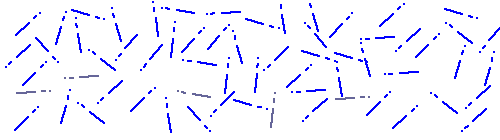Spacetime is then considered here to be occurring under motion-volumes f2 . d2 oriented in all directions.
To distinguish them from light waves, these motion-volumes are labelled present mo(ve)ment, which is interpreted here as being spacetime (the present moment) Reality.
• In this interpretation, the present mo(ve)ment is occurring as massless motions indefinitely renewing themselves throughout the entire Universe that is throughout spacetime.
• Because this present mo(ve)ment allows for light-speed, its motion-volumes are moving at light-speed written in capitals F2 . D2 parallelling physics' lightr speed squared capital C2.
• And to justify the above analogy, just as light speed is constant in the present movement, sound speed is constant in constant temperature air.
General relativity uncovers a fundamental characteristic of space and time, they make one; a surprising reality yet evident when considering spacetime motion volumes F2 . D2, in which space D2 and time F2 are irrevocably intertwined; when either one is modified a symetrical opposite modification of the other is occurring.
And to add to the confusion time F2 and space D2 are mathematically interchangeable, time may be called space and space called time the mathematics remain the same.
Being massless and occurring in all directions the present mo(ve)ment activity is temperature less, invisible and impalpable.
Spacetime is occurring as volumes of motion activity. Besides being self-driven that is active, the present mo(ve)ment is altogether finite (the duration of the present) and infinite (the present renews itself over and over).
Instead of just happening, our human concept of time is live, it is, along space, the cause of all happenings.

Particle matter and surrounding gravity. Take your pencil, lift it and release it. Your pencil is getting into motion, falling to the ground. Gravity, a Reality called gravitational acceleration in physics, is occurring as motion hence the word gravimotion,.
And the sensation we feel under our feet while standing up amounts to a stalled motion directed toward a center of gravity. Gravity is occurring under invisible stalled motion-volumes.
In gravimotion's interpretation, a particle matter is occurring at the image of gravity that is accelerated motions all directed toward the same center of gravity yet at a much higher density than gravity; gravity being now a byproduct of the matter it is surrounding.
At the origin of any material particle, 2 motion-volumes, within the present mo(ve)ment, collide head on, remain frozen against each other, then a great number of identical pairs aggregate and form a cluster around their collision center.
Both matter and surrounding gravity are organized alterations of the present mo(ve)ment; both occur as motion-volumes all directed toward a common center of gravity, yet stalled around it, in effect implementing our human concept of inertia.
In this process, the motion-volumes forming the present mo(ve)ment (spacetime) in which this interaction is occurring are pushed-out, and by reaction are oriented in the opposite direction toward the center, in the end establishing the gravimotion surrounding it.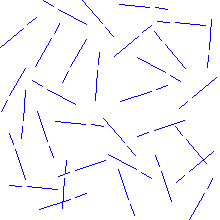In effect this nucleus creates in its surrounding a frozen acceleration of the present, amounting to the gravity surrounding any material entity.
This provides the physical mechanism acting behind matter / gravity inter dependency. The left illustration slows down at the end of each cycle to allow observation of the gravimotion surrounding the newly created particle.
Our human concepts of particle matter and gravity are, in gravimotion, integral part of the present mo(ve)ment; yet particle matter motion-volume pairs are compounded in such high density they cannot be penetrated by other particles of similar constitution.
The present mo(ve)ment's motion-volumes in blue are invisible to human eyes; the material subatomic particle popping out of it is represented in black.
In quantum mechanics, as already mentioned, particles made of energy pop haphazardly out of empty space; and to justify this emptiness (science's spacetime nonexistence), an antiparticle is popping out each time a particle does; in gravimotion, spacetime is not empty, it is instead occurring as motion-volumes out of which pop out particles configured as motion-volumes.
Material particles are popping out of the present, they are harnessing it and modifying it; neither creation nor loss of energy is occurring.

The particule's matter numerical expression.
But for the mass m, Einstein's m . C2 that is m . d2/t2 and a motion-volume f2 . d2 are mathematically identical.
• In gravimotion the term C2 = d2/t2 rewritten f2 . d2 takes over the factor m and is considered to represent the fabric of any material particle.
• the factor m is devalued to a pure number written #, a quantity having no unit.
• m . C2 is no longer an energy E but, as shown next, a particle occurring under stalled motion energy, which takes over our human concept of inert mass.
A cylindrical motion-volume length is an inverse time duration 1 / t squared, written f2; and the cross section d2 is a circle of radius r squared r2 = d2 / .When 2 motion-volumes collide head on as shown left, their lengths f2 get compacted against each other as shown right.
Such time compactions on both sides toward the center end up as 2 massless frozen accelerations or stalled motions that is the very fabric of gravity.
The pair is expressed here as the sum # . f2 . d2, in which the # = 2.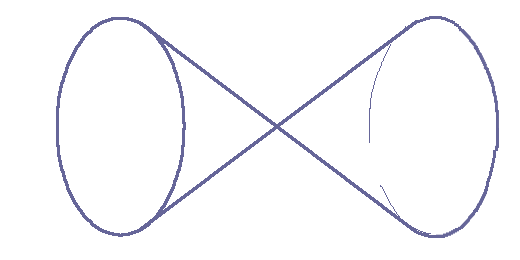When 5 or 100 or billions of pairs get together, to form a particle matter, each pair is in addition restricted toward the center as shown again on the right. Having identical bases and lengths as the compacted cylinders the cones volumes are 1/3 f2 . d2.
Note the product f2 . d2 considered in gravimotion is not an equation as E = mC2; that product f2 . d2 is energy on the move, a concept differing from physics' energy E.
Nevertheless this interpretation of matter is surprisingly echoing physics mathematics in which both matter energy mC2 and kinetic energy 1/2 mv2 involve squared speeds.
In General relativity gravity is part of space-time, here such affinity goes one step farther, space-time becomes matter's fabric.

Motion of Particle matter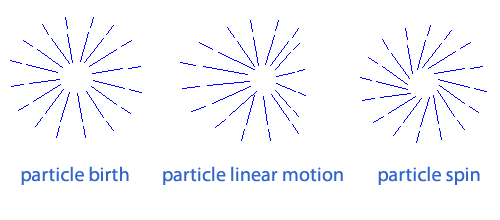An object is in motion anytime the motion-volumes pairs constituting it are set out of equilibrium; such an out of equilibrium state is provided by external motion entering it or overlapping it and creating within it a loss of equilibrium; this in accordance with physics' principle of “energy” conservation.
The illustration shows only the modification of the gravimotion surrounding the matter; an identical modification occurs within matter itself; setting out of equilibrium the center of gravity, which in physics is used to define the motion of the particle.
A spin constant motion just as a linear uniform motion does not require any additional incurring motion to occur; even though not mentioned in it, constant spin is integral part of Newton's first law. Turns, on the other hand, require out of line motion interaction to occur.
Mass, gravity and motion of mass are merged into a single entity. Gravimotion's (the gravity surrounding mass) modifications into linear and spinning motions are not part of physics.

Introducing swirling volumes The hand of the clock on the right side is rotating clockwise (CW).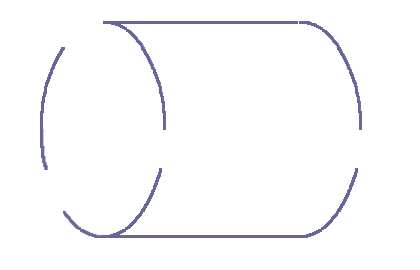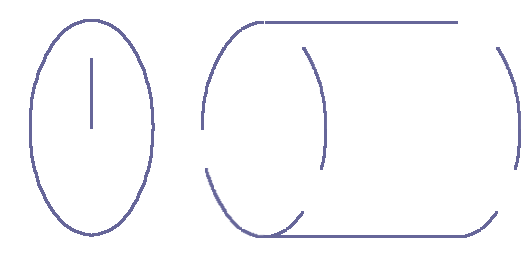And the motion-volume shown on its back exhibits an identical CW swirling; the latter is referred to as CW volume in the following.
On the left is a counter clockwise swirling volume referred to as CCW volume.
Now just as gravimotion's motion-volumes, these swirling volumes are not part of physics and do not exist in physics.
Please note that looking at the back of those 2 swirling volumes, the CW volume is seen as rotating CCW and the CCW as a CW; no matter our human thinking, these two are spinning in opposite directions when both are observed from the left.
Swirling and spinning refer to rotations, in the following the words swirling, spinning, circling, rotating, curling may be used.

Electrical Particles' fabric. In science physics there are positive and negative electric particles, which are made of quarks that are counted in thirds of electric charges; yet these electric charges are provided no physical fabric besides their positive or negative mathematical sign. The goal here is to unravel the physical structure occurring behind these so-called positive and negative mathematical signs.
1. As mentioned in "Particle matter and surrounding gravity" section, a material particle is occurring as a bunch of motion-volume pairs; the 2 motion-volumes of each pair butting at each other at a center of gravity.
2. In physics, electric particles of opposite sign attract each other; on the other hand two positive charges repel each other, as do two negative charges.
3. Matter's motion-volume pairs (item 1) now made swirling do implement the physical structure of an electric charge as defined in physics (item 2); a positive charge is physically implemented by CW volumes pairs, a negative charge by CCW volumes pairs, as described next.
Within the present mo(ve)ment (spacetime), the collision of 2 motion-volumes rushing against each other is ending in an inert stall creating a particle matter; yet the collided activity may in addition be stored under spinning motions, in such a way the particle is balanced, the process still complying with energy conservation.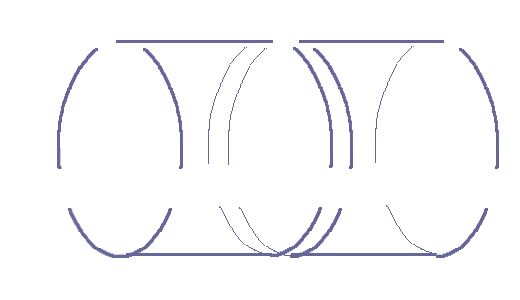As shown on the left, for an electrically negative particle such as an electron, the pairs collided on their respective CW front ends; now the spinning as viewed from either end of the pairs are circling CCW; the 2 motion-volumes find themselves entwined at the center into a strong CW CCW embrace, along science's electric positive negative strong attraction; and as shown right, both ends of a positive charge are observed spinning CW.
Just as in science in which the closer to the center of an electric charge the stronger the field, here the closer to the center of the rotating activity the higher its density and intensity;the farther from the center the weaker this rotating activity. This is represented at right in which the closer the swirling to the center the greater the activity per unit volume. With respect to the center, 2 symmetrical volumes are spinning in opposite directions, which is keeping the pair balanced unless modified by appropriate external motion.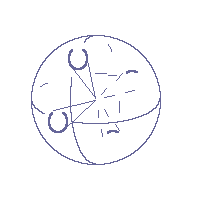A CW particle (positive particle ) is the assembly of a number of pairs; in actuality the particle's entire volume is filled with pairs; the individual pairs' volume restriction at center allow the whole particle final volume; on the left is the schematic of a CW particle in which only 2 CW pairs are shown.
CW and CCW volumes are, in gravimotion, the physical structures implementing particle's positive and negative mathematical signs.
Just as a particle matter is modifying its surrounding present mo(ve)mentn into gravimotion, an electrical particle spinning activity is modifying its surrounding present into spinning activity.

Electric field. Mutual interactions between the present and electrical CW and CCW particles are inducing CW and CCW curlings in this very present surrounding them, physically implementing physics' electric fields.The visible half sphere on the left is representing part of the present mo(ve)ment (spacetime) surrounding the above positive particle; it displays 8 spinning CW volumes corresponding to 8 CW pairs; yet unlike above their opposite symmetrical corresponding 8 CW volumes are not represented.
The present (spacetime) surrounding the particle is curled following the very particle's swirlings, its intensity is decreasing with distance in accordance to the increase of the circling volumes cross sections constituting them.
And just as physics' positive charges are behaving, the clockwise-curlings surrounding CW particles are repulsing other CW particles surroundings; on the other hand CW and CCW particles surroundings work like hand in glove.
Gravimotion (a particle's surrounding gravity) remains oriented toward the center of gravity, whether the particle surroundings exhibit electrical curlings or not.

Electrical conductor Matter is composed of atoms each made of a nucleus around which are circling CCW particles of type electrons; the outer circling electrons are called here peripheral electrons riding peripheral trajectories; in metals such as copper these peripheral trajectories are oriented in all directions and the peripheral electrons are free to jump between the atoms peripheral trajectories; when prompted by an external electrical activity all these electrons move in orderly direction within the wire along the orientation of the activity (along time); copper is a conductor; per definition a conductor offers free passage to a flow of electrons called electric current.
Within a battery, a number of CW particles are kept away (forming the positive pole) from a number of CCW particles (the battery negative pole); viewed from the outside that amounts to 2 stalled activities (physics' positive and negative electric field), which when connected through an external conductor wire of electricity engenders an electric current.

Magnetic activity surrounding any electrical current. In science physics any electrical current, that is a number of electrons moving in a unified motion, implements in its surrounding a magnetic field. The right hand rule provides the direction of the magnetic field. Set your right thumb in the direction of the current, the wrapping of your other fingers are in the direction of the magnetic field.
In gravimotion the surrounding of the wire that is spacetime called the present mo(ve)ment is occurring as a sea of uninhibited motions; the current in the wire modifies this surrounding present motions into circling motions round the wire.

Magnets. Iron and nickel are conductors that can be turned into magnets. Placing an iron rod within a strong CW (North) CCW (South) magnetic activity modifies its atoms' peripheral trajectories.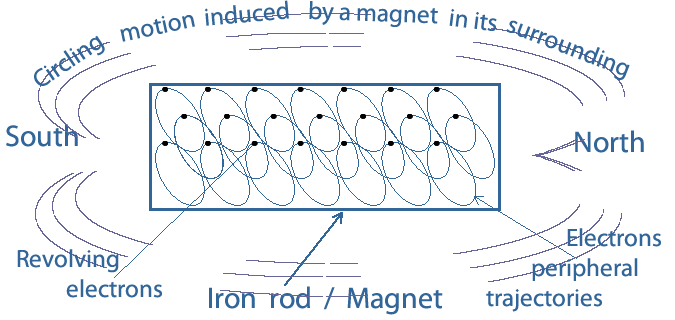The external CW CCW present mo(ve)ment revolutions penetrate the rod and have the effect to orient the atom's peripheral trajectories parallel to each other as shown on the left and the peripheral electrons are forced to be circling in concert.
Once the external strong North South magnetic activity has been removed, the peripheral electrons continue circling in tune with each other, which keeps their peripheral trajectories aligned, the whole amounting to a circling electrical current enforcing the rod's own magnetic activity. CW and CCW circlings on ends are enforcing the North and South poles of the new magnet,
This magnet in turn is implementing circular motions in its surroundings (physics' magnetic field).
At the image of gravitational matter which is re-orienting the present (spacetime) surrounding it, magnets are re-orienting the very motions of the present mo(ve)ment surrounding them yet in a different way.

Electromagnetic waves and photons In the following in order to simplify the language, only a small spectrum of the electromagnetic waves that are light waves and their photons are considered.

The photon's numerical expression In science a photon is energy provided by Planck's equation
E = h . f .
h is Planck's constant defined as h = kg . 6 10-34 meter2 / second; in gravimotion the kg is just a number and its unit is replaced by 1, Plank's constant is written:
1 . 6 10-34 . 1 meter2 / 1 second   or   6 10-34 . 1 meter2 / 1 second
f is the frequency characterising the photon considered and mathematically defined as a number (#) of cycles per second written here f = # / 1 second; as their letters do, the frequency f is turning out to be an invere time t.
The photon's h . f is then written:
(6 10-34 . 1 meter2 / 1 second) . f   and   (6 10-34 . 1 meter2 / 1 second) . # / 1 second
or:
# . 6 10-34 . 1 meter2 / 1 second2         equivalent to         # . 6 10-34 . 1 f2 . 1 d2
Here the number # is precisely the frequency and 6 10-34 . 1 f2 . 1 d2 is interpreted as being the fundamental motion-volume boldly called Plank's motion-volume and written with capitals to express so:
6 10-34 . F2 . D2.
F being the inverse of a time unit and D the unit distance in the SI system of units, their representation being capitalized at the image of Eisntein's famous capital C2.
Light's photon ends up being the product of a fundamental motion-volume by a # proportional to the frequency, which fits Plank's extraordinary expression h . f.
In gravimotion, a photon so called particle is occurring as a number of f2 . d2 motion-volumes just as the particle matter is.
Devaluing our human concept of mass, C2 appearing in Einstein mC2 and Plank's h . f are equivalent, amounting to a unified interpretation of Nature.

The photons' inner mechanism. Beneath these succinct mathematics, is an intricate inner mechanism.
In this animation, single photons are crossing your screen's surface, popping out of it one after another; each is occurring as a motion-volume defined as a cylinder having for base the surface area of the top circle and for length a duration coinciding to the the full revolution of the radius, precisely lasting the inverse of the wave's period.
Beware, while based on science physics, a photons' activity or motion-volume departs greatly from science's particle photon. In science the photon's frequency is energy; when considering the wave rather than the particle, such energy is occurring as fields graphically represented with two electric and electromagnetic sinusoids offset 900 degrees with respect to each other; when either one of these two fields is null the other is at an absolute maximum, as such a photon can be represented as done here as two simultaneous sine and cosine events.
The animation below coincides with a specific polarized light, such as a blue photon for instance, but represented at a much lower frequency.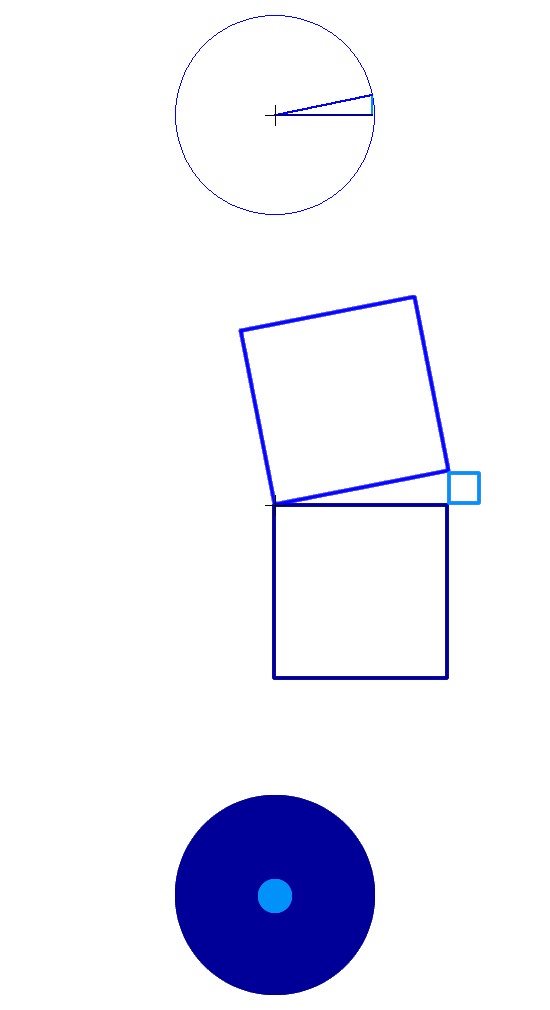In the top animation the plain blue revolving radius of length r determines successive angles; the cosines of these angles (the dark blue horizontal lines) coincide to the electric field variation; the sines (the light blue vertical lines) coincide to the magnetic field; the dark and light blue line that is the electric and magnetic fields are at 90 degrees with respect to each other just as in science physics. Usually, in mathematics, the sine is represented coincident to the vertical axle; here the sine lines are shifted to the end of the cosines.

In the second animation, squares are attached to these 3; according to Pythagorean's theorem the plain blue radius squared: r2 is at all times equal to the horizontal cosine squared plus the vertical sine squared; here the radius, cosine and sine lengths are multiplied by √ Π (read square root of pie) so the area of the squares attached to the revolving radius (in plain blue) are at all times unchanged and precisely equal to the surface of above circle; the light and dark blue squares areas only are varying. And as can be seen when either of these two squares' area is maximum the other is null.

In gravimotion the electric-magnetic duality occurs as follows: the electric component (dark blue squares) below the horizontal to start with, while decreasing is transferring its energy to the magnetic (light blue squares) above the horizontal, which as a consequence is increasing; when this magnetic component reaches a maximum it rebounds within the common allocated motion-volume, and is restituting back the energy to the electric component; a full cycle is involving 4 transfers.

The third animation illustrates this interaction; the dark blue surface (cosine squared or electric field) and the lightblue surface (sine squared or magnetic field) are mutually interacting with each other over a full period.
Physics' representation of a light wave that is 2 offset by 90 degrees sine waves are interpreted here as a constant sum r2 = cos2() + sin2() and its correlative Π r2 = Π cos2() + Π sin2(), a constant which brings to mind light's constant speed.
Next is a photon interpretation occurring under gravimotion's 3 dimensional spacetime.
Note this brings to mind Hermann Minkowski's much more sophisticated s2, space-time-interval, which is using Pythagoreans' four dimensional theorem: s2 = x2  + y2  + z2 - c2t2, in which x, y, z are space coordinates, c a constant speed and t a time. A space-time-interval being actually the length of a straight line joining two specific points defined by sa2 = xa2  + ya2  + za2 - c2ta2 and sb2 = xb2  + yb2  + zb2 - c2tb2.

The photons' motion-volume constituent. Physics' photon energy is E = h f in which the frequency f is lasting a specific time duration. The goal here is to define the motion-volume constituent (MVC), which while renewing itself along the present ends up as the full photon motion-volume (PMV) now coinciding to one cycle of the wave.
Please note the MVC is not part of physics' science, neither is identifying the photon energy to a cycle of the wave, as that goes against light duality.
Physics' light wave is made of 2 sine waves offset 90 degrees with respect to each other; in the table below, one cycle of these 2 sine waves is represented.
The number of MVCs constituting one cycle can be chosen at will; 24 MVCs have been chosen in the following PMV interpretation, each MVC time duration lasting the time it takes the above radius to rotate 15 degrees.
The table below only provides the values of the first 12 MVCs from 1 to 180 degrees as the other 12 MVCs from 181 to 360 degrees are the mirror image of it.

The odd number rows 3, 5 up to 25 show in column A the radius angles 150, 300, 450 up to 1800 defining the ends of each of the 12 first MVCs; in the same odd rows, the sine() and sine2() values of these angles appear in columns C and D respectively; the 900 offset sine(+90) and sine2(+90) values appear in columns F and G labeled as such; the 3600 value appearing in row# 1 column A coincides to the end of the previous cycle not part of the considered cycle.
Most importantly, the sine2() and sine2(+90) columns D and G are the algebraic expressions of the dark and light blue squares in the above illustration..
As can be seen in column J their sum is always equal to 1 that is reflecting above radius squared constant surface.

The evenly numbered rows 2. 4, 6 ... 24, printed in green color concern the MVCs proper. Column B labelled "MVC span" shows the 15 degrees spans coinciding to the 24 constituents presently chosen to constitute a full 360 degrees rotation; in column E, labelled Sin2() span, is the difference between the 2 Sin2() values appearing in the previous columns D upper and lower rows; in column H is the difference between the 2 Sin2(+90) values appearing in the previous column G upper and lower rows; these E and H columns are representing time durations (or spans) and surfaces both as such labeled span.
For instance the number 0.1831 appearing in column E row# 4 is the difference between the 2 values 0.0669 and 0.25 appearing in column D, rows# 3 and 5 .

Remarkably, the 2 values appearing in even rows, column E and H are almost equal; yet they must be considered so as the sum of the above dark and light blue squares animation remains unchanged; when one decreases the other increase by the same amount; in gravimotion interpretation and as explained next, these 2 identical values are respectively attributed to the MVCs' cross sections and time durations.

 Col  A Radius angledegrees B MVC spanin degrees C Sin() D Sin2() E Sin2() span F Sin(+90) G Sin2(+90) H Sin2(+90)span JSin2()+ Sin2(+90) MVCsSin2()span / Sin2(+90) span 1 360 0 0 1 1 1 2 15 0.0669 0.0669 1 3 15 0.2588 0.0669 0.9659 0.9329 1 4 15 0.1831 0.183 1 5 30 0.5 0.25 0.8660 0.7499 1 6 15 0.25 0.2499 1 7 45 0.7071 0.5 0.7071 0.5 1 8 15 0.2499 0.25 1 9 60 0.8660 0.7499 0.5 0.25 1 10 15 0.183 0.183 1 11 75 0.9659 0.9329 0.2588 0.0669 1 12 15 0.0671 0.0669 1 13 90 1 1 0 0 1 14 15 0.0671 0.0669 1 15 105 0.9659 0.9329 -0.2588 0.0669 1 16 15 0.183 0.1831 1 17 120 0.8660 0.7499 -0.5 0.25 1 18 15 0.2499 0.25 1 19 135 0.7071 0.5 -0.7071 0.5 1 20 15 0.25 0.2499 1 21 150 0.5 0.25 -0.8660 0.7499 1 22 15 0.1831 0.183 1 23 165 0.2588 0.0669 -0.9659 0.9329 1 24 15 0.0669 0.0669 1 25 180 0 0 -1 1 1 Radius angledegrees Col  A MVC spanin degrees B Sin()  C Sin2()  D Sin2() span  E Sin(+90)  F Sin2(+90)  G Sin2(+90span  H Sin2()+ Sin2(+90)J Sin2()span / Sin2(+90) spanMVCs

The ratios sine2() span over sine2(+90) span appearing in the MVCs column (last column on right), all equal to 1, amount each at multiplying sine2() span by the inverse of sine2(+90) span.
Now here, the sine2 spans are interpreted as being the cross sections of the successive individual MVCs; and the sine(+90)2 spans are interpreted as being the time durations (acceleration or deceleration) it takes the radius, in the present chosen case, to rotate 15 degrees; the product of the 2 that is a MVC in each case transmitting the full amount of energy determined by the frequency f.
The energy occurring during one PMV cycle, proportional to f, is renewing itself during its own cycle; it is occurring over a number of time durations MVCs, each transmitting the full energy of the photon rather than transmitting lower energies the sum of which amounting to the whole.
The transmitting of energy in a continuous way coincides to the constant speed of light, even during the photon's life, which is not evident considering the ups and downs of physics 2 sine waves representation.
While both involve motion, the difference between gravimotion's motion-volume (MV) and Minkowski's space-time-interval (STI) is that the MV is the fabric of all together space-time, matter and electromagnetic waves, STI is not.

The present mo(ve)ment (spacetime) numerics. In the absence of electromagnetic wave energy, the above plain blue radiuses rather than oscillating in harmony are oriented in all directions implementing the present mo(ve)ment (spacetime) as introduced in Spacetime is given the name present mo(ve)ment section.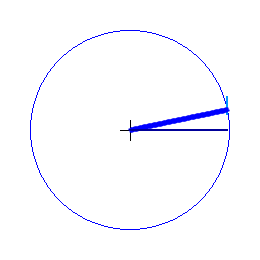The motion-volumes making the present are defined as:
66 10-33 . 1 inverse-second2 . 1 meter2
This basic motion-volume ends up as being the fundamental fabric of spacetime, the fabric of material particles and electromagnetic waves.
The present is self propelling, an activity constantly renewing itself, a fact we are all witnessing at each instant of our lives.
Even though advocated by Einstein this spacetime-oneness is difficult to imagine as most of us human beings think that time is running independently of anything else and more so independently of space.
The way to answer is to note that the present only is real; to morrow's world even the world of the next second does not exist; and yesterday's entire Universe along that of last second does no longer exist; the past just as the future are never in force; everything that surrounds us in space, along the whole physical Universe, is constantly renewing itself along the present.
This present constant renewing has fundamental consequences; first it keeps the identity of things alive allowing them not to disappear in between what we call two successive time instants; then it allows evolution; these two fundamentals in turn are mandating their cause, which is constant renewed duration.
That contradicts quantum uncertainty, just as all other branches of physics, which aere based on cause and effect, do contradict uncertainty.

Regarding the analogy spacetime / air temperature used here in the first place
Per definition inertia (inert mass), as is defined the particle matter in science, cannot be acting as kinetic energy. And to corroborate, the photon is proof that energy on the move is occurring independently of inert mass.
In our human quest for unification, rather than identifying the electromagnetic wave to a particle as done in science, we may as well identify the particle matter to motion as done in gravimotion.
Yet to do so we must devalue our concept of mass to a number having no unit; a situation incompatible with Einstein's equation E = mC2, kinetic energy E = 1/2 mv2 and Boltzmann temperature T = m v2 / 3k; as unit wise the term E would be thrown off balance.
Yet, based on the units of measurement, the following shows a temperature T is equal to a speed squared v2.
The Calorie, an old unit of energy, has been redefined as being equivalent to 4.2 Joules. 1 calorie (thermo chemical) = 4.184 joule exactly.
1 calorie = 4.184 joule (1)
The Joule, a unit of energy equivalent to a force multiplied by distance, is defined as 1 Joule = 1 Newton x 1 meter.
1 calorie = 4.184 x 1 Newton x 1 meter (2)
The Newton, a unit of force equivalent to the acceleration of matter, is defined as 1 Newton = 1 kilogram of matter accelerated at the rate of 1 meter per second per second.
1 calorie = 4.184 x 1 Kg x (1 meter/ 1 sec sec) x 1 meter (3)
or:
1 calorie = 4.184 x 1 Kg x 1 meter2 / (1 sec)2 But the calorie a unit of heat (heat being a specific form of energy), is defined as being the heat added to one gram of water to raise it from 14.5 to 15,5 degree Celsius.
1o Celsius x 1 gram = 4.184 x 1 Kg x (1 meter)2 / (1 sec)2(4)
or:
1o Celsius x 1 gram = 4.184 x 1000 gram x (1 meter)2 / (1 sec)2(5)
which can be rewritten:
1o Celsius x 1 gram = 4,184 gram x (1 meter)2 / (1 sec)2(6)
and dividing each side by one gram:
1o Celsius = 4,184 (1 meter)2 / (1 sec)2 (7)
Or within a constant :
To = v2(8)
A temperature change of 1 degree, is equivalent to a speed squared of 1 meter2 / 1 second2; and a # T of degrees is a # of 1 meter2 / 1 second2.
Written in terms of motion-volumes as # of inverse-second2 . 1 meter2.
This justifies, in this mind of mine, the assumption that spacetime is occurring at the image of the air molecules movements and called the the present mo(ve)ment.

Copyright © 2023 Henri Salles. You have the permission to reproduce, print, distribute and post the contents of this website, provided you mention the link: https://gravimotion.com. Contact henriJMsalles[at]gmail.com.